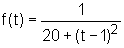Ch 1. Limits Multimedia Engineering Math Limit of aSequence Limit of aFunction LimitLaws Continuity RateChange
 Chapter 1. Limits 2. Derivatives I 3. Derivatives II 4. Mean Value 5. Curve Sketching 6. Integrals 7. Inverse Functions 8. Integration Tech. 9. Integrate App. 10. Parametric Eqs. 11. Polar Coord. 12. Series Appendix Basic Math Units Search eBooks Dynamics Fluids Math Mechanics Statics Thermodynamics Author(s): Hengzhong Wen Chean Chin Ngo Meirong Huang Kurt Gramoll ©Kurt GramollMATHEMATICS - CASE STUDY IntroductionDriving Process Jim and Jerry are working on a new tpye of spaceport material. According to their experiment data, the thermal conductivity coefficient of this material is a function of the temperature. Because this material will be used in extremely low and high temperature, they want to find this coefficient at specific points. The unit of t is oc and the unit for f(t) is W/(m-oc). What is known: Function isQuestions What is the heat transfer coefficient of this material at -∞, 1, +∞? Approach The limit of this function at -∞, 1, +∞ is the coefficient of this material.